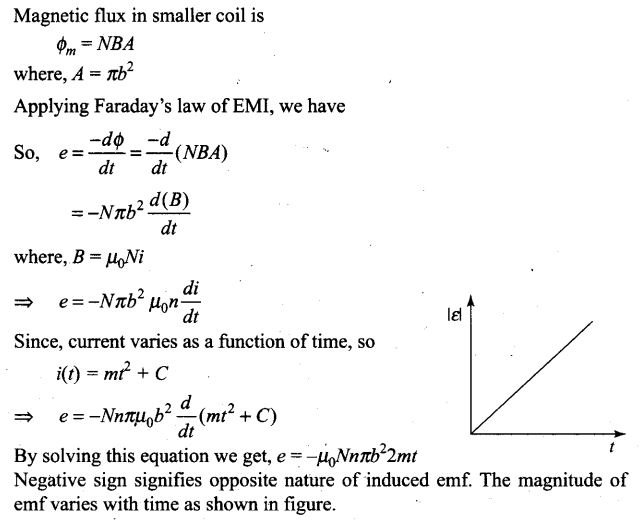PHP error in Ad Inserter block 1 - Block 1
Warning: Undefined variable \$link

# Electromagnetic Induction NCERT Exemplar Problems Solutions Physics

## NCERT Exemplar Problems Class 12 Physics Chapter 6 Electromagnetic Induction

Multiple Choice Questions (MCQs)
Single Correct Answer Type
Question 1.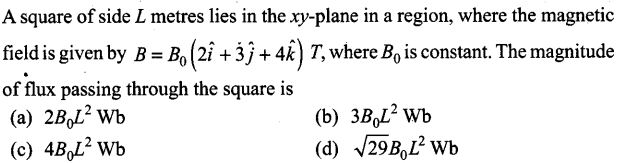Solution: (c)
Key concept: Magnetic flux is defined as the total number of magnetic lines of force passing normally through an area placed in a magnetic field and is equal to the magnetic flux linked with that area.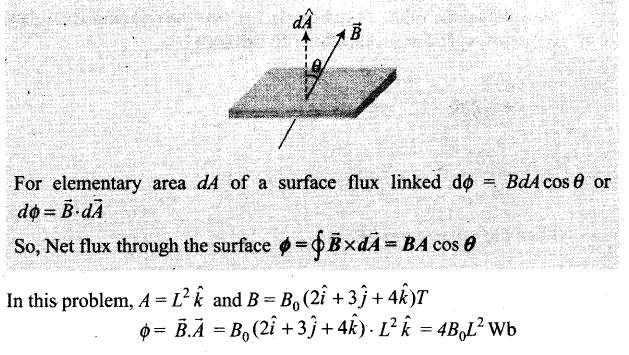Question 2.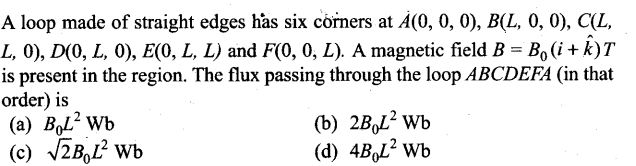Solution: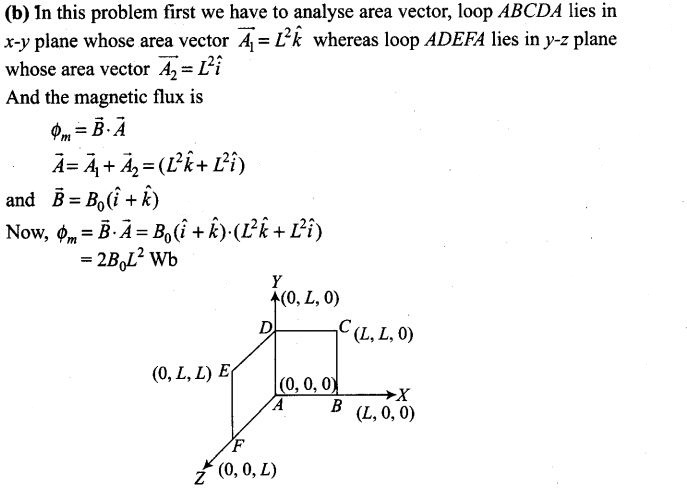Question 3. A cylindrical bar magnet is Rotated about its axis. A wire is connected from the axis and is made to touch the cylindrical surface through a contact. Then,
(a) a direct current flows in the ammeter A
(b) no current flows through the ammeter A
(c) an alternating sinusoidal current flows through the ammeter A with a time period 2π /ω
(d) a time varying non-sinusoidal current flows through the ammeter A
Solution: (b)
Key concept: The phenomenon of electromagnetic induction is used in this problem. Whenever the number of magnetic lines of force (magnetic flux) passing through a circuit changes (or a moving conductor cuts the magnetic flux) an emf is produced in the circuit (or emf induces across the ends of the conductor) is called induced emf. The induced emf persists only as long as there is a change or cutting of flux.
When cylindrical bar magnet is rotated about its axis, no change in flux linked with the circuit takes place, consequently no emf induces and hence, no current flows through the ammeter A. Hence the ammeter shows no deflection.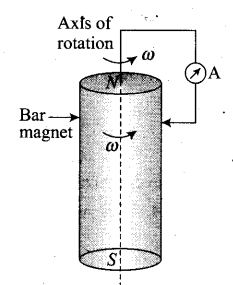Question 4. There are two coils A and B as shown in figure. A current starts flowing in B as shown, when A is moved towards B and stops when A stops moving. The current in A is counter clockwise. B is kept stationary when A moves. We can infer that
(a) there is a constant current in the clockwise direction in A.
(b) there is a varying current in A.
(c) there is no current in A.
(d) there is a constant current in the counter clockwise direction in A.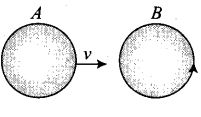Solution: (d)
Key concept: Due to variation in the flux linked with coil B an emf will be induced in coil B.
Current in coil B becomes zero when coil A stops moving, it is possible only if the current in coil A is constant. If the current in coil A would be variable, there must be some changing flux and then there must be an induced emf. Hence an induced current will be in coil B even when coil A is not moving.

Question 5. Same as problem 4 except the coil A is made to rotate about a vertical axis (figure). No current flows in B if A is at rest. The current in coil A, when the current in B (at t = 0) is counter-clockwise and the coil A is as shown at this instant, t = 0, is
(a) constant current clockwise.
(b) varying current clockwise.
(c) varying current counter clockwise.
(d) constant current counter clockwise.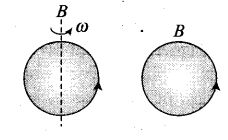Solution: (a)
Key concept: In this problem, the Lenz’s law is applicable so let us introduce Lenz’s law first. .
Lenz’s law gives the direction of induced emf/induced current. According to this law, the direction of induced emf or current in a circuit is such as to oppose the cause that produces it. This law is based upon law of conservation of energy.
When the current in coil B (at t= 0) is counter-clockwise and the coil A is considered above it. The counter clockwise flow of the current in coil B is equivalent to north pole of magnet and magnetic field lines are eliminating upward to coil A. When coil A starts rotating at t = 0, the current in A is constant along clockwise direction by Lenz’s rule.

Question 6. The self inductance L of a solenoid of length l and area of cross-section A, with a fixed number of turns N increases as
(a) l and A increase (b) l decreases and A increases
(c) l increases and A decreases (d) both l and A decrease
Solution: (b)
Key concept: The self inductance L of a solenoid depends on various factor like geometry and magnetic permeability of the core material.
L = μr μ0 n2 Al
where, n = N/l (no. of turns per unit length)
1. No. of turns: Larger the number of turns in solenoid, larger is its self inductance.
2. Area of cross section: Larger the area of cross section of the solenoid, larger is its self inductance.
3. Permeability of the core material. The self inductance of a solenoid increases μr times if it is wound over an iron core of relative permeability μr.
The long solenoid of cross-sectional area A and length l, having A turns, filled inside of the solenoid with a material of relative permeability (e.g., soft iron, which has a high value of relative permeability) then its self inductance is L = μrμ0 N2 A/l
So, the self inductance L of a solenoid increases as l decreases and A increases because L is directly proportional to area and inversely proportional to length.
Important point: The self and mutual inductance of capacitance and resistance depend on the geometry of the devices as well as permittivity/ permeability of the medium.

One or More Than One Correct Answer Type
Question 7. A metal plate is getting heated. It can be because
(a) a direct current is passing through the plate
(b) it is placed in a time varying magnetic field
(c) it is placed in a space varying magnetic field, but does not vary with time
(d) a current (either direct or alternating) is passing through the plate
Solution: (a, b, c)
Key concept: Eddy Current: When a changing magnetic flux is applied to a bulk piece of conducting material, then circulating currents called eddy currents are induced in the material. Because the resistance of the bulk conductor is usually low, eddy currents often have large magnitudes and heat up the conductor.
(1) These are circulating currents like eddies in water.
(2) Experimental concept is given by Focault, hence also named as “Focault current”.
(3) The production of eddy currents in a metallic block leads to the loss of electric energy in the form of heat.
(4) By lamination, slotting processes, the resistance path for circulation of eddy current increases, resulting into weakening them and also reducing losses causes by them.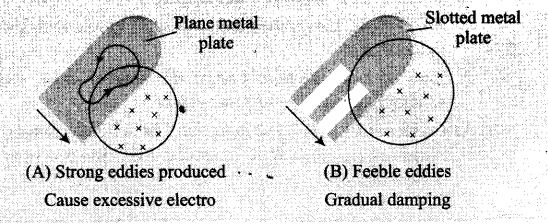A metal plate is getting heated when a DC or AC current is passed through the plate, known as heating effect of current. This current (called eddy current) is induced in the plate when a metal plate is subjected to a time varying magnetic field, i.e., the magnetic flux linked with the plate changes and eddy currents comes into existence which make the plate hot.

Question 8. An emf is produced in a coil, which is not connected to an external voltage source. This can be due to
(a) the coil being in a time varying magnetic field
(b) the coil moving in a time varying magnetic field
(c) the coil moving in a constant magnetic field
(d) the coil is stationary in external spatially varying magnetic field, which does not change with time
Solution: (a, b, c)
Key concept: As we know whenever the number of magnetic lines of force (magnetic flux) passing through a circuit changes, an emf is produced in the circuit called induced emf. The induced emf persists only as long as there is a change or cutting of flux.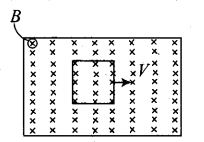In this problem, magnetic flux linked with the isolated coil changes when the coil is placed in the region of a time varying magnetic field, the coil moving in a constant magnetic field or in time varying magnetic field.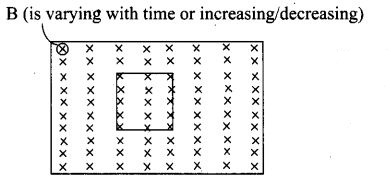Question 9. The mutual inductance M12 of coil 1 with respect to coil 2
(a) increases when they are brought nearer
(b) depends on the current passing through the coils
(c) increases when one of them is rotated about an axis
(d) is the same as M21 of coil 2 with respect to coil 1
Solution: (a, d)
Key concept: Mutual Induction: Whenever the current passing through a coil or circuit changes, the magnetic flux linked with a neighbouring coil or circuit will also change. Hence an emf will be induced in the neighbouring coil or circuit. This phenomenon is called ‘mutual induction’.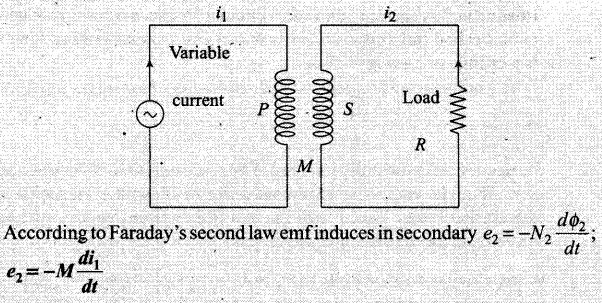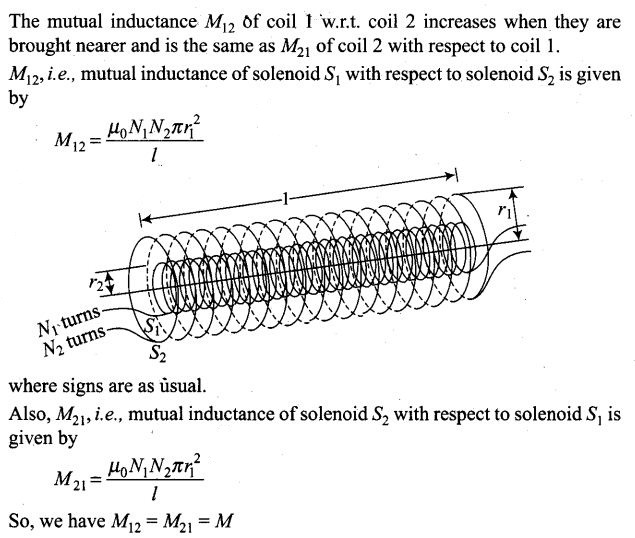Question 10. A circular coil expands radially in a region of magnetic field and no electromotive force is produced in the coil. This can be because
(a) the magnetic field is constant
(b) the magnetic field is in the same plane as the circular coil and it may or may not vary
(c) the magnetic field has a perpendicular (to the plane of the coil) component whose magnitude is decreasing suitably
(d) there is a constant magnetic field in the perpendicular (to the plane of the coil) direction
Solution: (b, c)
Key concept: As we know whenever the number of magnetic lines of force (magnetic flux) passing through a circuit changes an emf is produced in the circuit called induced emf. The induced emf persists only as long as there is a change or cutting of flux.
The induced emf is given by rate of change of magnetic flux linked with the circuit, i.e., e = -dФ/dt
According to the problem there is no electromotive force produced in the coil. Then the various arrangement are to be thought of in such a way that the magnetic flux linked with the coil does not change even if the coil is placed and expanded in magnetic field.
When circular coil expands radially in a region of magnetic field such that the magnetic field is in the same plane as the circular coil or we can say that direction of magnetic field is perpendicular to the direction of area (increasing) so that their dot product is always zero and hence change in magnetic flux is also zero.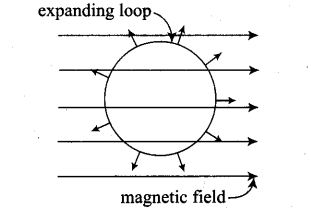Or
The magnetic field has a perpendicular (to the plane of the coil) component whose-magnitude is decreasing suitably in such a way that the dot product of magnetic field and surface area of plane of coil remain constant at every instant.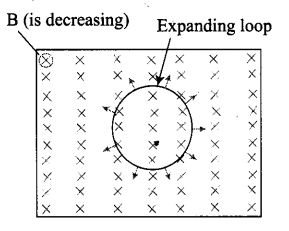Very Short Answer Type Questions
Question 11. Consider a magnet surrounded by a wire, with an on/off switch S (figure). If the switch is thrown from the off position (open circuit) to the on position (closed circuit), will a current flow in the circuit? Explain.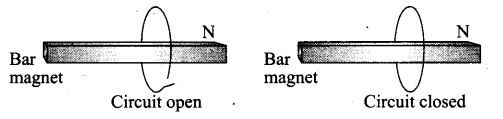Solution: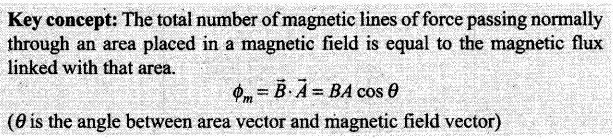Whenever the number of magnetic lines of force (magnetic flux) passing through a circuit changes an emf is produced in the circuit called induced emf. The induced emf persists only as long as there is a change or cutting of flux. The induced emf is given by rate of change of magnetic flux linked with the circuit i.e, e= – dФ/dt . so flux linked will change when either magnetic field, area or the angle between B and A changes.
If the switch is closed, the circuit will complete. But to induce emf in the circuit, we need:
(i) a changing magnetic field, but the bar magnet is stationary so it is not possible in this situation.
(ii) A changing area, which is also not possible because area is also constant as coil is not expanding or compressed.
(iii) Angle between B bar and A bar changes, which is also not possible in this situation because orientation of bar magnet and coil is fixed.
Thus, no change in magnetic flux linked with coil occur, hence no electromotive force is induced in the coil and hence no current will flow in the circuit.

Question 12. A wire in the form of a tightly wound solenoid is connected to a DC source, and carries a current. If the coil is stretched so that there are gaps between successive elements of the spiral coil, will the current increase or decrease? Explain.
Solution:
Key concept: Lenz ‘s Law:
This law gives the direction of induced emf/induced current. According to this law, the direction of induced emf or current in a circuit is such as to oppose the cause that produces it. This law is based upon the law of conservation of energy.
(1) When AT-pole of a bar magnet moves towards the coil, the flux associated with the loop increases and an emf is induced in it. Since the circuit of loop is closed, induced current also flows in it.
(2) Cause of this induced current, is approach of north pole and therefore to oppose the cause, i.e., to repel the approaching north pole, the induced current in loop is in such a direction so that the front face of loop behaves as north pole. Therefore induced current as seen by observer O is in anticlockwise direction (figure).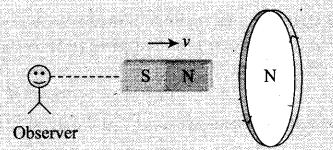According to the given situation as the coil is stretched so that there are gaps between successive elements of the spiral coil, i.e., the wires are pulled apart which lead to the flux leak through the gaps. According to Lenz’s law, the emf induced in these spirals must oppose this decrease in magnetic flux, which can be done by an increase in current. So, the current will increase.

Question 13. A solenoid is connected to a battery so that a steady current flows through it. If an iron core is inserted into the solenoid, will the current increase or decrease? Explain.
Solution: This problem is based on Lenz’s law and according to this law, the direction of induced emf or current in a circuit is such as to oppose the cause that produces it.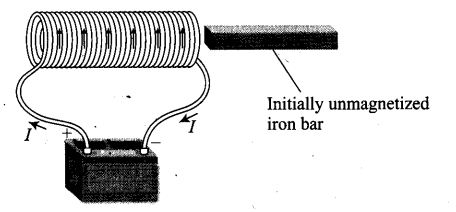When the iron core is inserted in the current carrying solenoid, the magnetic field increases due to the magnetisation of iron core and hence the flux increases.
So, the emf induced in the coil must oppose this increase in flux, so the current induced in the coil in such a direction that it will oppose the increasing magnetic field which can be done by making decrease in current. So, the current will decrease.

Question 14. Consider a metal ring kept oft the top of a fixed solenoid (say on a cardboard) (figure). The centre of the ring coincides with the axis of the solenoid. If the current is suddenly switched on, the metal ring jumps up. Explain.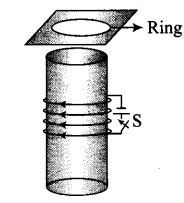Solution: This problem is based on Lenz’s law and according to this law, the direction of induced emf or current in a circuit is such as to oppose the cause that produces it.
Initially there is no flux linked with the ring or we can say that initially flux through the ring is zero. When the switch is closed current start flowing in the circuit, magnetic flux is linked through the ring. Thus increase in flux takes place. According to Lenz’s law, this increase will be resisted and this can happen if the ring moves away from the solenoid.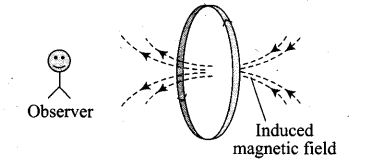This happen because the flux increases will cause an anticlockwise current (as seen from the top in the ring in figure.), i.e., opposite direction to that in the solenoid.
This makes the same sense of flow of current in the ring (when viewed from the bottom of the ring) and solenoid forming same magnetic pole in front of each other. Hence, they will repel each other and the ring will move upward.

Question 15. Consider a metal ring kept (supported by a cardboard) on the top of a fixed solenoid carrying a current I (see figure of Question 14). The centre of the ring coincides with the axis of the solenoid. If the current in the solenoid is switched off, what will happen to the ring?
Solution: This problem is based on Lenz’s law and according to this law, the direction of induced emf or current in a circuit is such as to oppose the cause that produces it.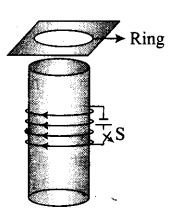When the switch is opened, current in the circuit of solenoid stops flowing. Initially there is some magnetic flux linked with the solenoid and now if current in the circuit stops, the magnetic flux falls to zero or we can say that magnetic flux linked through the ring decreases. According to Lenz’s law, this decrease in flux will be opposed and the ring experiences downward force towards the solenoid.
This happen because the current i decrease will cause a clockwise current (as seen from the top in the ring in figure) to increase the decreasing flux. This can be done if the direction of induced magnetic field is same as that of solenoid. This makes the opposite sense of flow of current in the ring (when viewed from the bottom of the ring) and solenoid forming opposite magnetic pole in front of each other.
Hence, they will -attract each other but as ring is placed at the cardboard it could not be able to move downward.

Question 16. Consider a metallic pipe with ah inner radius of 1 cm. If a cylindrical bar magnet of radius 0.8 cm is dropped through the pipe, it takes more time to come down than it takes for a similar un-magnetised cylindrical iron bar dropped through the metallic pipe. Explain.
Solution: Key concept: Lem’s Law.
This law gives the direction of induced emf/induced current. According to this law, the direction of induced emf or current in a circuit is such as to oppose the cause that produces it. This law is based upon the law of conservation of energy.
Eddy Current. When a changing magnetic flux is applied to a bulk piece of conducting material, then circulating currents called eddy currents are induced in the material. Because the resistance of the bulk conductor is usually low, eddy currents often have large magnitudes and heat up the conductor.
When a cylindrical bar magnet is dropped through the metallic pipe flux linked with the cylinder changes and consequently eddy currents are produced in the metallic pipe. According to Lenz’s law, these currents will oppose the motion of the magnet, which is the cause of induction.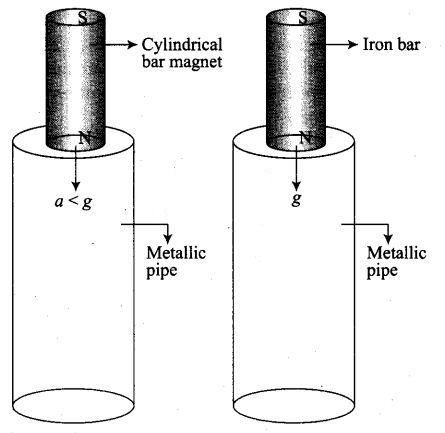Therefore, magnet’s downward acceleration will be less than the acceleration due to gravity g. On the other hand, an un-magnetised iron bar will not produce eddy currents and will fall with acceleration due to gravity g.
Thus, the magnet will take more time to come down than it takes for a similar un-magnetised cylindrical iron bar dropped through the metallic pipe, so, magnetised magnet takes more time.

Short Answer Type Questions
Question 17. A magnetic field in a certain region is given by B = B0 cos (ωt) k and a coil of radius a with resistance R is placed in the x-y plane with its centre at the origin in the magnetic field (figure). Find the magnitude and the direction of the current at (a, 0, 0) at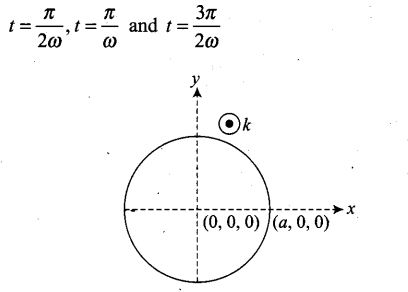Solution:
Key concept:
(1) First law: When ever the number of magnetic lines of force(magnetic flux) passing through a circuit changes, an emf is produced in the circuit called induced emf. The induced emf persists only as long as there is a change or cutting of flux.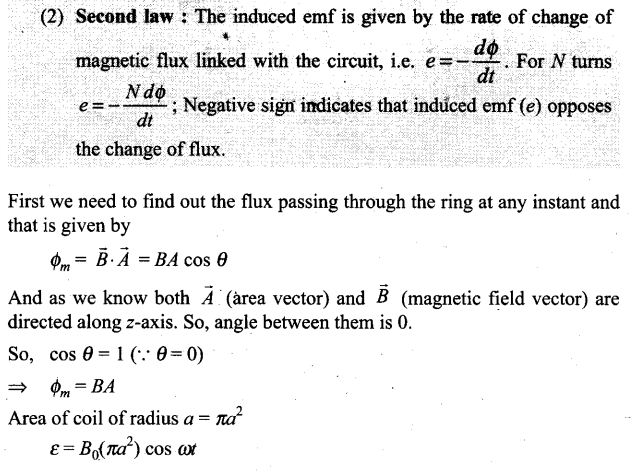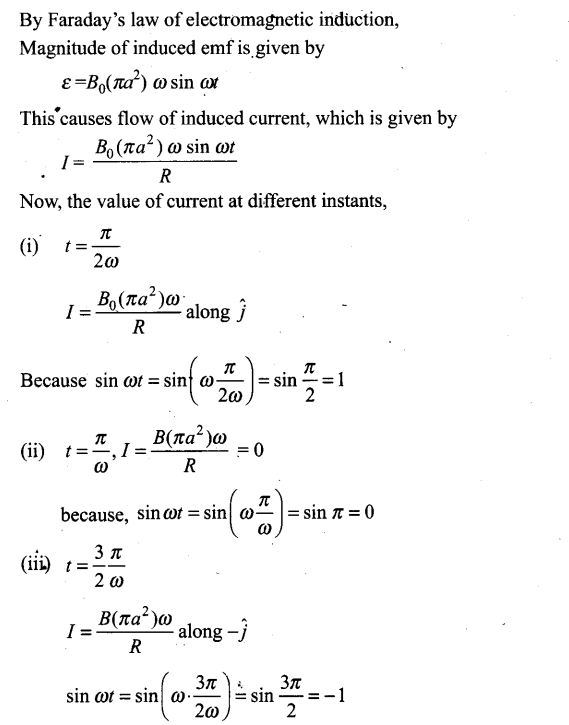Question 18. Consider a closed loop C in a magnetic field (figure). The flux passing through the loop is defined by choosing a surface whose edge coincides with the loop and using the formula Ф = B1dA1, B2dA2 …. Now, if we choose two different surfaces S1 and S2 having C as their edge, would we get the same answer for flux. Justify your answer.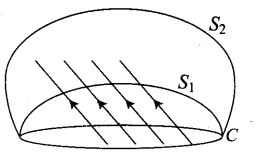Solution: We would get the same answer for magnetic flux. Let us discuss its reason in detail. The magnetic flux linked with the surface can be considered as the number of magnetic field lines passing through the surface. So, let dФ = B.dA represents magnetic lines in an area A to B.
Magnetic field cannot end or start in space, this property of magnetic field lines based upon the concept of continuity. Therefore the number of lines passing through surface S1 must be the same as the number of lines passing through the surface S2. Therefore, in both the cases we get the same magnetic flux.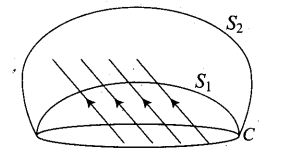Important point: Magnetic field lines can neither be originated nor be destroyed in space. This property is based on the concept of continuity.

Question 19. Find the current in the wire for the configuration shown in figure. Wire PQ has negligible resistance. B, the magnetic field is coming out of the paper. θ is a fixed angle made by PQ travelling smoothly over two conducting parallel wires separated by a distance d.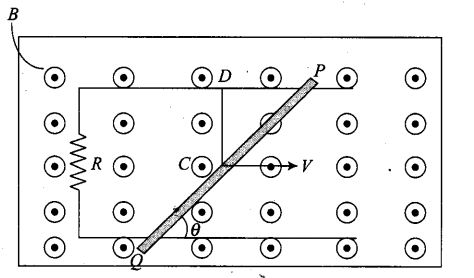Solution:
Key concept: This problem is based upon the motional emf.
Consider a conducting rod of length l moving with a uniform velocity v perpendicular to a uniform magnetic field B bar, directed into the plane of the paper. Let the rod be moving to the right as shown in figure. The conducting electrons also move to the right as they are trapped within the rod.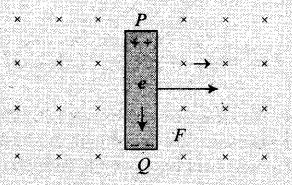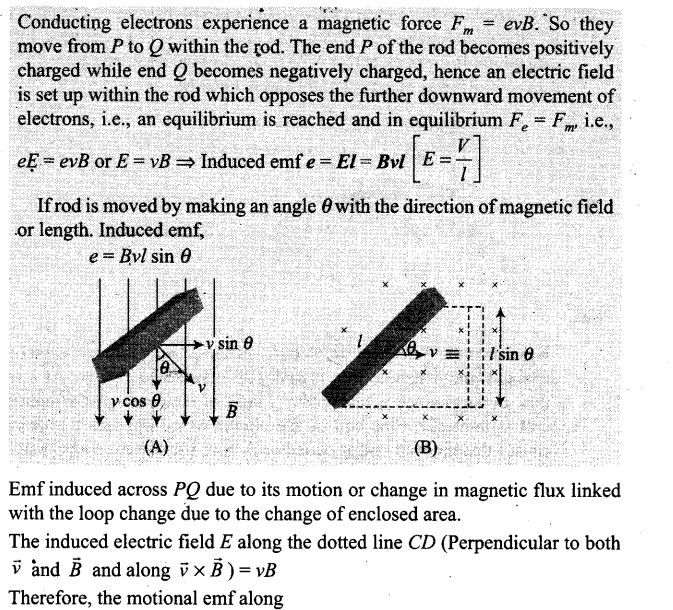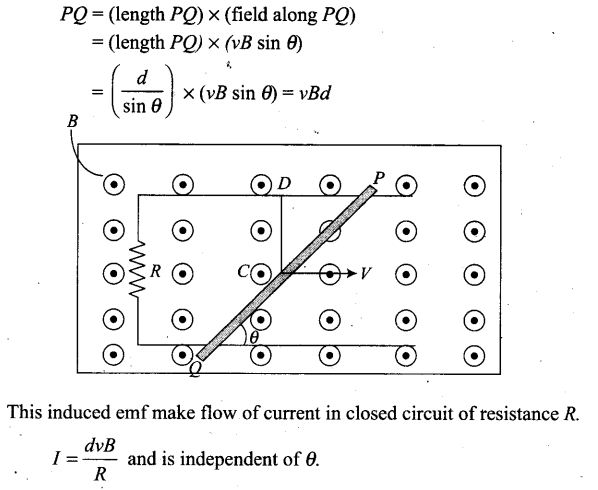Question 20. A (current versus time) graph of the current passing through a solenoid is shown in figure. For which time is the back electromotive force (u) a maximum. If the back emf at t = 3 s is e, find the back emf at t = 7 s, 15 s and 40 s. OA, AB and BC are straight line segments.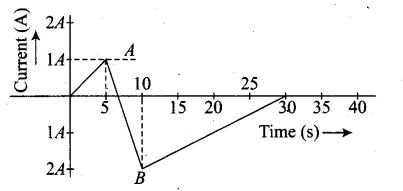Solution:
Key concept: Whenever the electric current passing through a coil or circuit changes, the magnetic flux linked with it will also change. As a result of this, in accordance with’Faraday’s laws of electromagnetic induction, an emf is induced in the coil or the circuit which opposes the change that causes this induced emf is called back emf, the current so produced in the coil is called induced current. The induced emf is given by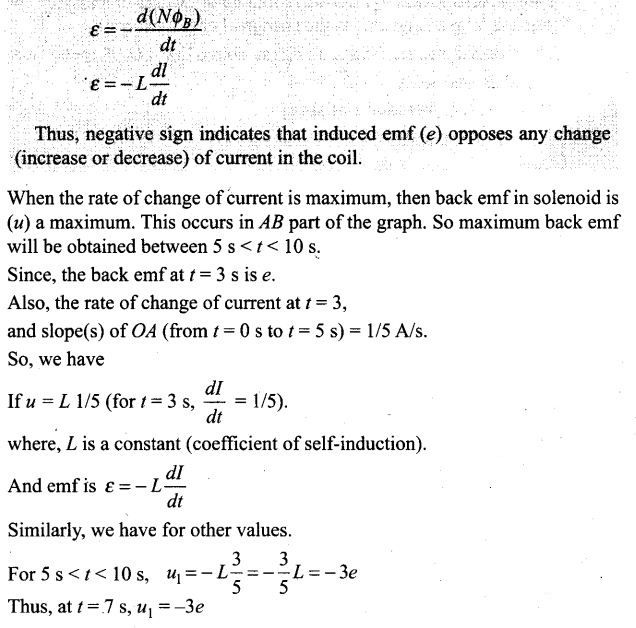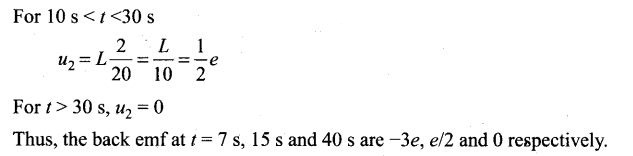Question 21. There are two coils A and B separated by some distance. If a current of 2 A flows through A, a magnetic flux of 10-2 Wb passes through B (no current through B). If no current passes through A and a current of 1A passes through B, what is the flux through A ?
Solution: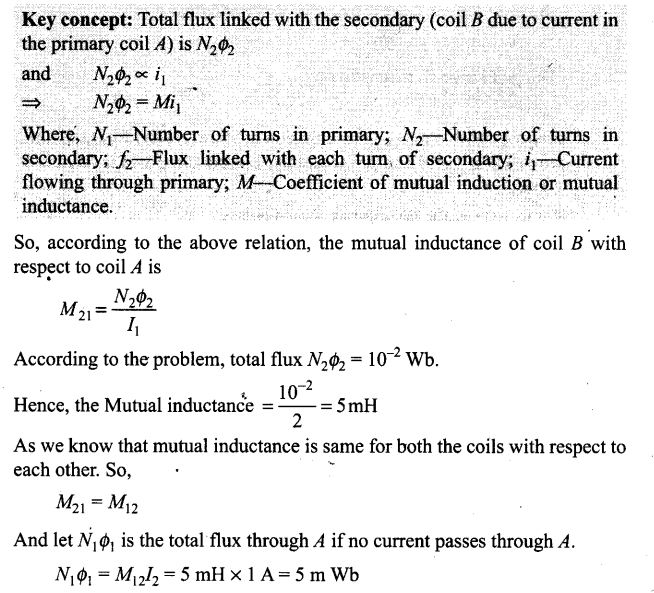Long Answer Type Questions
Question 22. A magnetic field B = B0 sin (ωt)k covers a large region where a wire AB slides smoothly over two parallel conductors separated by a distance d (figure). The wires are in the x-y plane. The wire AB (of length d) has resistance R and the parallel wires have negligible resistance. If AB is moving with velocity v, what is the current in the circuit. What is the force needed to keep the wire moving at constant velocity?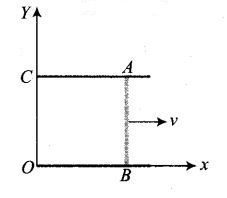Solution: Key concept: In this problem the emf induced across AB is motional emf due to its motion, and emf induced by change in magnetic flux linked with the loop change due to change of magnetic field.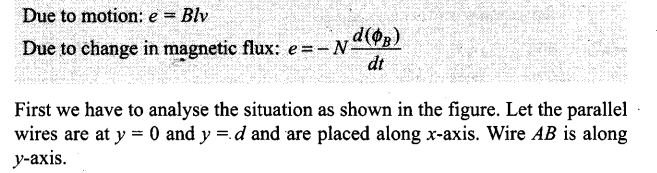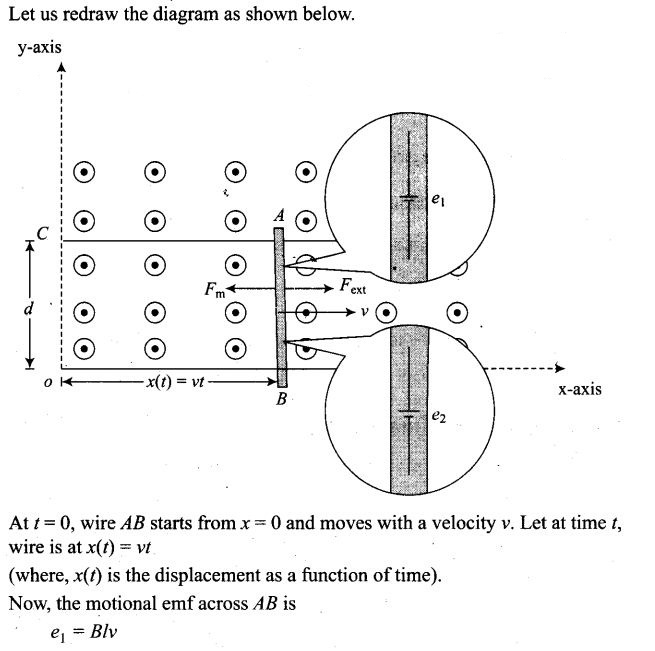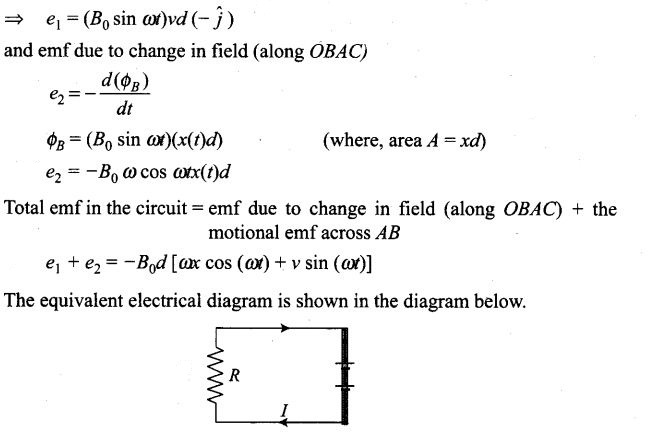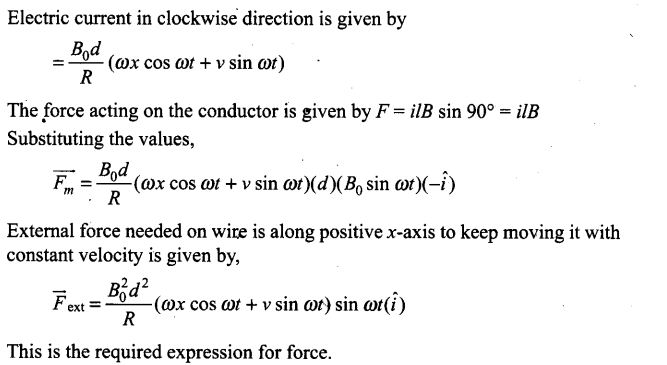Question 23. A conducting wire XY of mass m and negligible resistance slides smoothly on two parallel conducting wires as shown in figure. The closed circuit has a resistance R due to AC. AB and CD are perfect conductors. There is a magnetic field B = B(t) k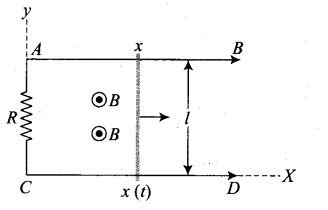(i) Write down an equation for the acceleration of the wire XY
(ii) If B is independent of time, obtain v(t), assuming v(0) = u0
(iii) For (ii), show that the decrease in kinetic energy of XY equals the heat lost in.
Solution: First we have to analyse the situation as shown in the figure. Let the parallel wires are at y = 0 and y = L and are placed along x-axis. Wire XY is along y-axis.
At t = 0, wire AB starts from x = 0 and moves with a velocity v. Let at time t, wire is at x(t) = vt.
(where, x(t) is the displacement as a function of time).
Let us redraw the diagram as shown below.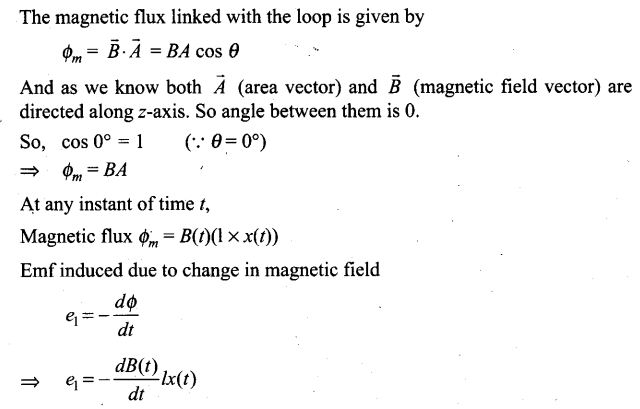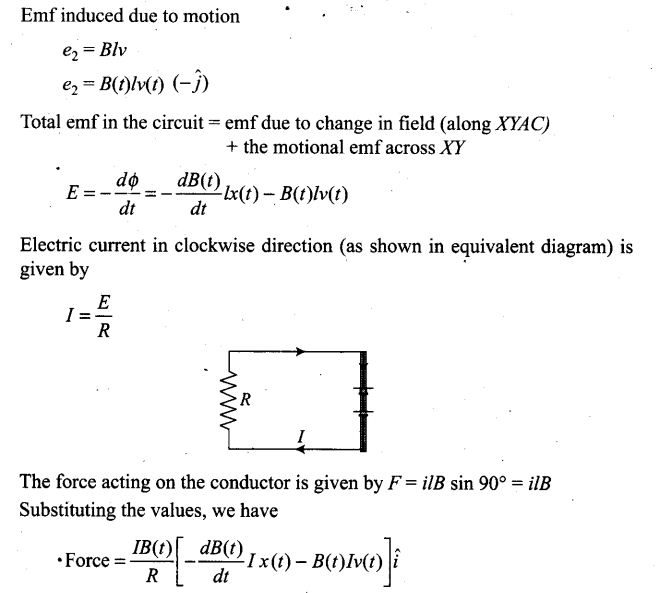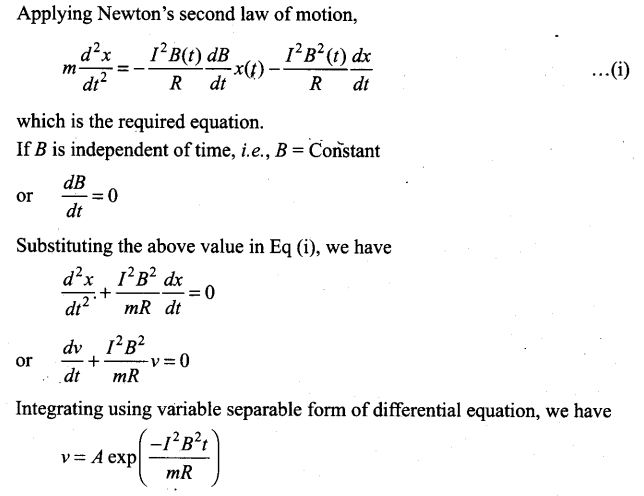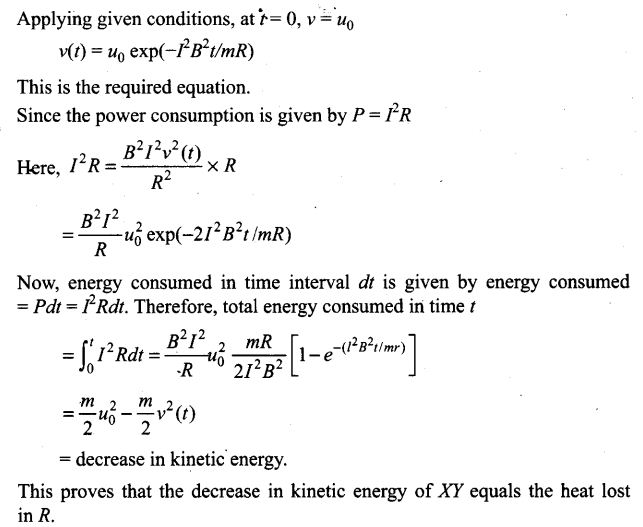Question 24. ODBAC is a fixed rectangular conductor of negligible resistance (CO is not connected) and OP is a conductor which rotates clockwise with an angular velocity ω (figure). The entire system is in a uniform magnetic field B whose direction is along the normal to the surface of the rectangular conductor A’BDC. The conductor OP is in electric contact with ABDC. The rotating conductor has a resistance of λ per unit length. Find the current in the rotating conductor, as it rotates by 180°.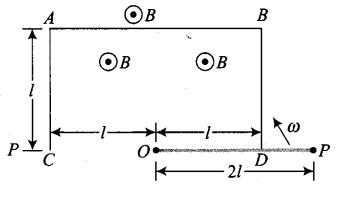Solution: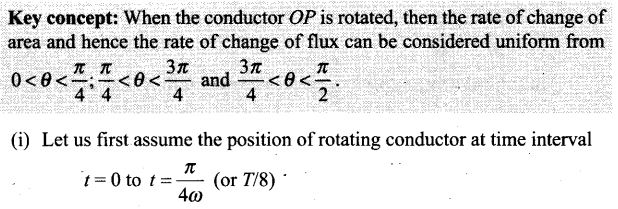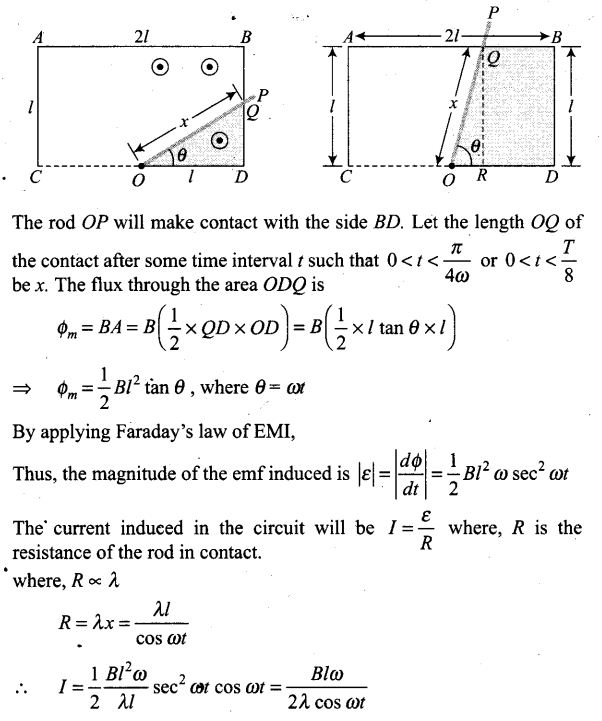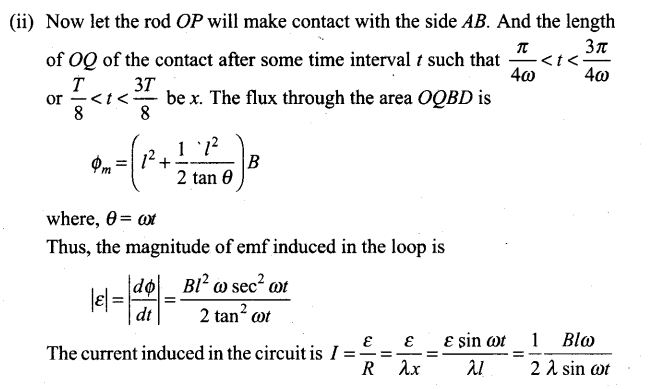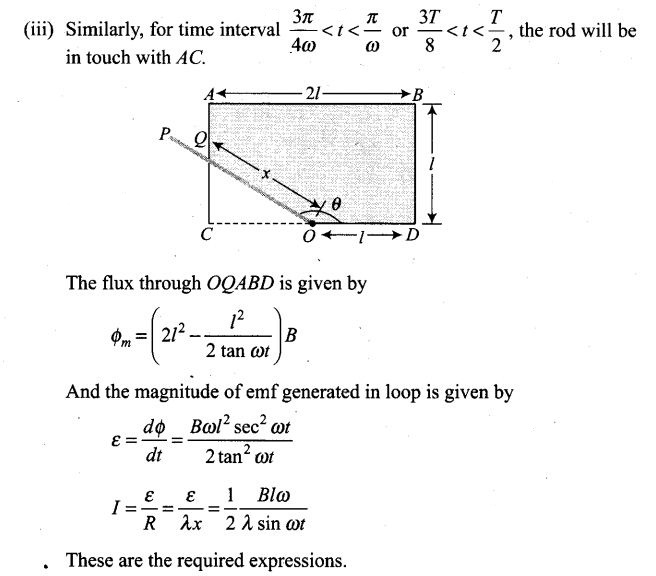Question 25. Consider an infinitely long wire carrying a current I(t), with dI/dt=λ =constant. Find the current produced in the rectangular loop of wire ABCD if its resistance is R (figure).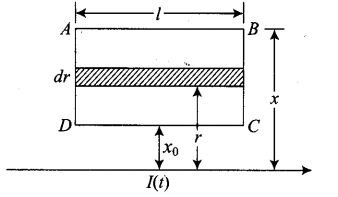Solution: To approach these types of problems integration is very useful to find the total magnetic flux linked with the loop.
Let us first consider an elementary strip of length l and width dr at a distance r from an infinite long current carrying wire. The magnetic field at strip due to current carrying wire is given by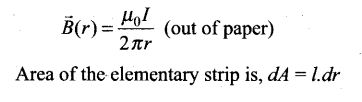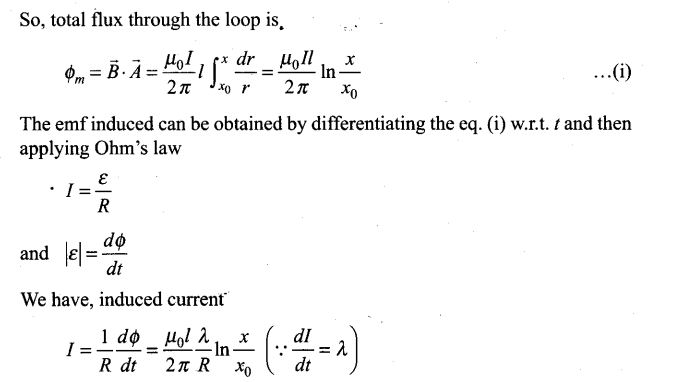Question 26. A rectangular loop of wire ABCD is kept close to an infinitely long wire carrying a current I(t) = I0(I – t/T) for 0 ≤ t ≤ T and I(0) = 0 for t >T(figure). Find the total charge passing through a given point in the loop, in time T. The resistance of the loop is R.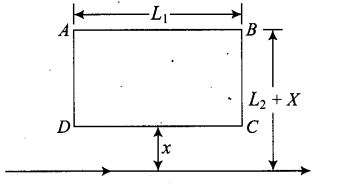Solution: To find the charge that passes through the circuit first we have to find the relation between instantaneous current and instantaneous magnetic flux linked with it. The emf induced can be obtained by differentiating the expression of magnetic flux linked w.r.t. t and then applying Ohm’s law, we get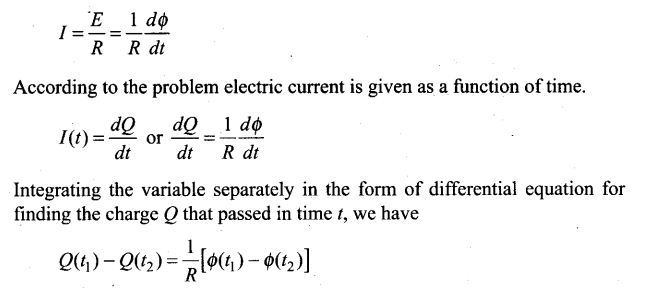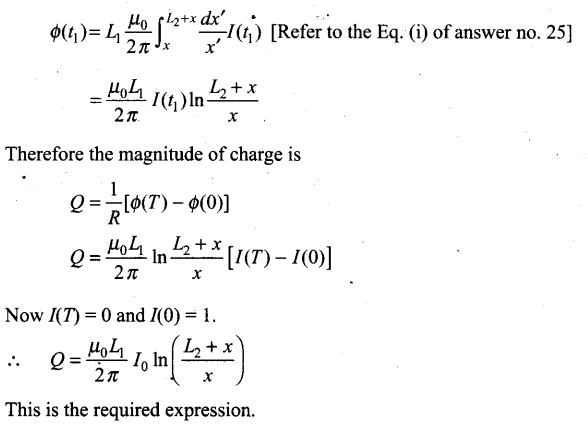Question 27. A magnetic field B is confined to a region r ≤ a and points out of the paper (the z-axis), r = 0 being the centre of the circular region. A charged ring (charge = Q) of radius b, b> a and mass m lies in the x-y plane with its centre at the origin. The ring is free to rotate and is at rest. The magnetic field is brought to zero in time Δt. Find the angular velocity ω of the ring after the field vanishes.
Solution:
Key concept: According to the law of EMI, when magnetic field changes in the circuit, then magnetic flux linked with the circuit also changes and this changing magnetic flux leads to an induced emf in the circuit. Here, magnetic field decreases which causes induced emf and hence, electric field around the ring. The torque experienced by the ring produces change in angular momentum.
As the magnetic field is brought to zero in time At, the magnetic flux linked with the ring also reduces from maximum to zero. This, in turn, induces an emf in ring as discussed above. The induces emf causes the induced electric field E around the ring.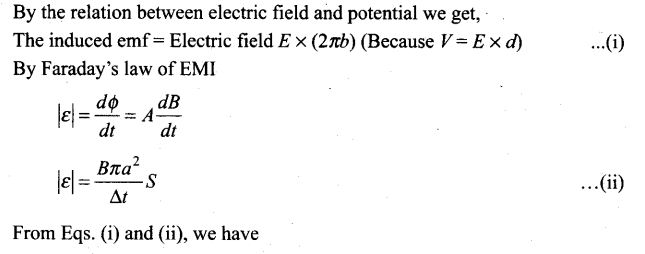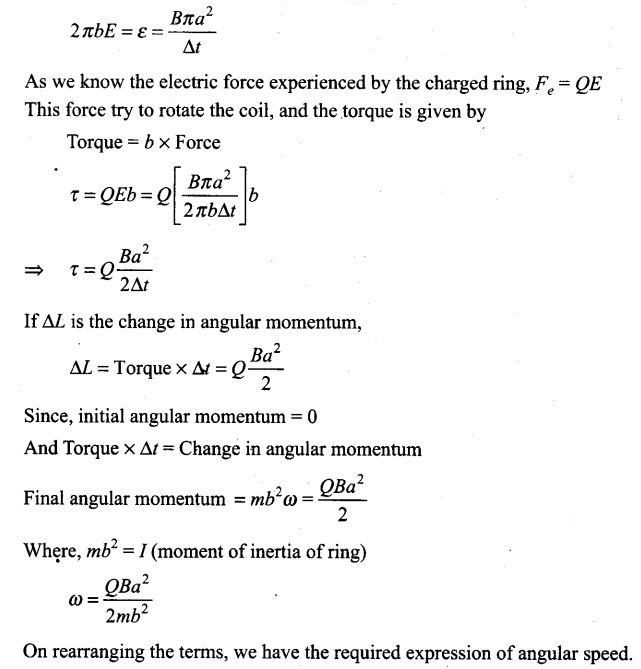Question 28. A rod of mass m and resistance R slides smoothly over two parallel perfectly conducting wires kept sloping at an angle θ with respect to the horizontal (figure). The circuit is closed through a perfect conductor at the top. There is a constant magnetic field B along the vertical direction. If the rod is initially at rest, find the velocity of the rod as a function of time.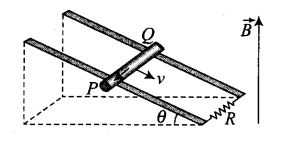Solution:
Let us first divide the magnetic field in the components one is along the inclined plane = B sin θ and other component of magnetic field is perpendicular the plane =B cos θ
Now, the conductor moves with speed v perpendicular to B cos θ, component of magnetic field. This causes motional emf across two ends of rod, which is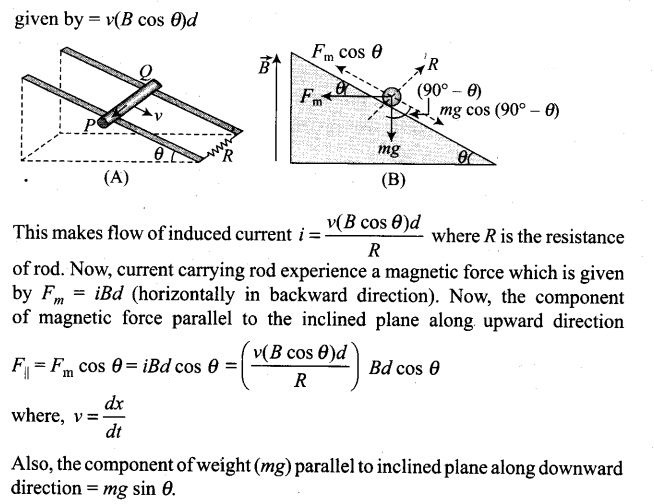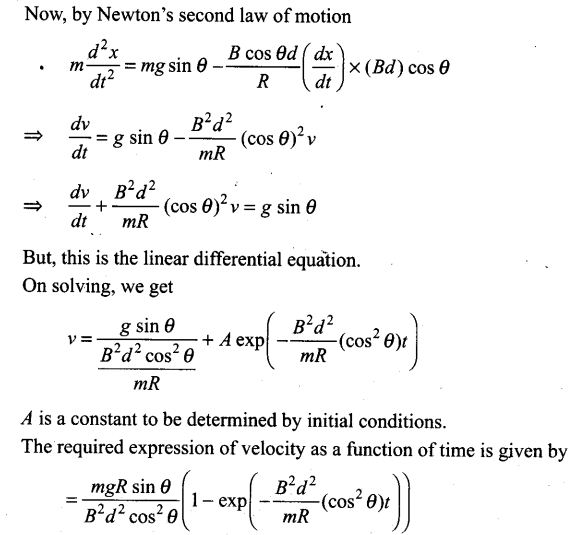Question 29. Find the current in the sliding rod AB (resistance = R) for the arrangement shown in figure. B is constant and is out of the paper. Parallel wires have no resistance, v is constant. Switch S is closed at time t = 0.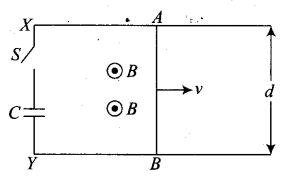Solution: This is the similar problem as we discussed above. Here, a conductor of length d moves with speed v, perpendicular to the magnetic field B as shown in figure. Due to this a motional emf is induced across two ends of rod (e = vBd). Since, switch S is closed at time t = 0, capacitor is charged by this potential difference. Let Q(t) be the charge on the capacitor and current flows from A to B.
Now, the induced current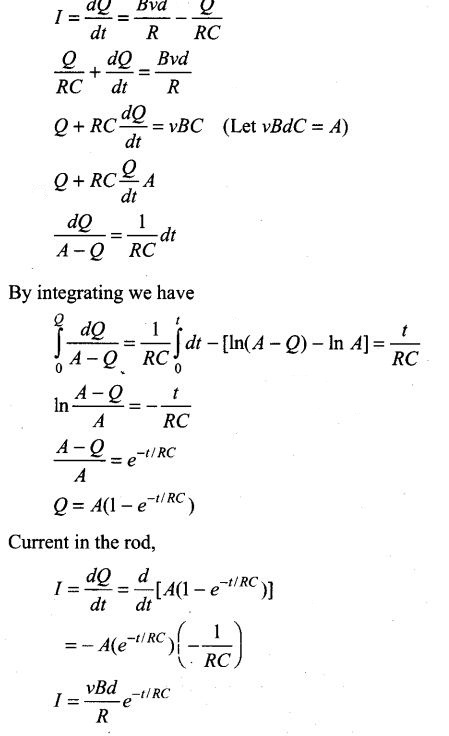Question 30. Find the current in the sliding cod AB (resistance = R) for the arrangement shown in figure. B is constant and is out of the paper. Parallel wires have no resistance, v is constant. Switch S is closed at time t = 0.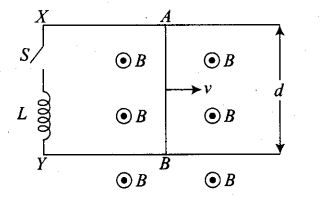Solution: This is the similar problem as we discussed above. Here, a conductor of length d moves with speed v, perpendicular to magnetic field B as shown in figure. Due to this an motional emf is induced across two ends of rod (e = vBd). Since, switch S is closed at time t = 0. current start growing in inductor by the potential difference due to motional emf.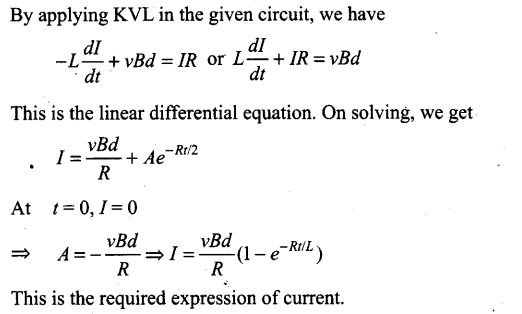Question 31. A metallic ring of mass m and radius l (ring being horizontal) is falling under gravity in a region having a magnetic- field. If z is the vertical direction, the z-component of magnetic field is Bz = B0 (1+ λz). If R is the resistance of the ring and if the ring falls with a velocity v, find the energy lost in the resistance. If the ring has reached a constant velocity, use the conservation of energy to determine v in terms of m, B, λ and acceleration due to gravity g.
Solution: In this problem a relation is established between induced current, power lost and velocity acquired by freely falling ring.
The magnetic flux linked with the metallic ring of mass m and radius l ring being horizontal falling under gravity in a region having a magnetic field whose z-component of magnetic field is Bz = B0(1 + λz) is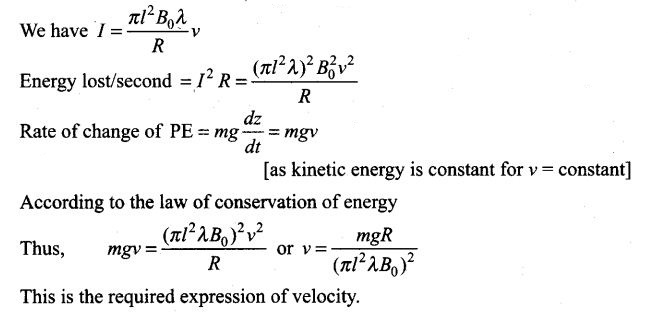Question 32. A long solenoid S has n turns per metre, with radius a. At the centre of this coil, we place a smaller coil of N turns and radius b (where b < a). If the current in the solenoid increases linearly with time, what is the induced emf appearing in the smaller coil. Plot a graph showing nature of variation in emf, if current varies as a function of mt2 + C.
Solution:
Key concept: Magnetic field due to a solenoid is given by, B = μ0ni where signs are as usual.
In this problem the current is varying with time. Due to this an emf is induced in the coil of radius b.